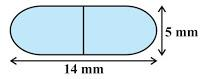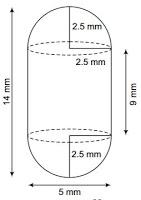# A medicine capsule is in the shape of a cylinder with two hemispheres stuck to each of its ends. The length of the entire capsule is 14 mm and the diameter of the capsule is 5 mm. Find its surface area.The capsule comprises two hemispheres and one cylinder is shown in the figureGiven

The diameter of the capsule = 5 mm

∴ Radius = 5/2 = 2.5 mm

Now, the length of the capsule = 14 mm

The length of the cylinder = 14-(2.5+2.5) = 9 mm

Find out

We have to find out the Surface area of the cylinder

Solution

The surface area of cylinder=C.S. An of cylinder+ C.S.A of 2 Hemisphere

=2πrh+2πr2*2

=2πr(h+2r)

On substituting the known values we get

=2*22/7*5/2(9+2*5/2)

=110/7(9+5)

=110/7*14

=220mm2

The surface area of the cylinder =220mm2(1)(0)